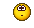I wrote:

> I seem to recall Matthew had trouble proving the composite of conjoints is the conjoint of the composite:
>
> $\check{FG} \stackrel{?}{=} \check{F} \check{G} .$
>
> I don't even know if this is true! It would seem strangely asymmetrical for this to be false.

Matthew wrote:

> It is asymmetrical! I don't think this is true in general.

> Instead, we have

> $\check{G F} = \check{F} \check{G}$

Oh, duh! Right!This is not asymmetrical in any 'bad' sense; it's just the natural thing. Let \$$\mathbf{Cat}_{\mathcal{V}}\$$ be the category with

* \$$\mathcal{V}\$$-enriched categories as objects

* \$$\mathcal{V}\$$-enriched functors as morphisms

and let \$$\mathbf{Prof}_{\mathcal{V}}\$$ be the category with

* \$$\mathcal{V}\$$-enriched categories as objects

* \$$\mathcal{V}\$$-enriched profunctors as morphisms.

Then taking companions gives an inclusion of \$$\mathbf{Cat}\_{\mathcal{V}}\$$ in \$$\mathbf{Prof}\_{\mathcal{V}}\$$ while taking conjoints gives an inclusion of \$$\mathbf{Cat}\_{\mathcal{V}}^{\text{op}}\$$ in \$$\mathbf{Prof}_{\mathcal{V}}\$$.

In other words, less technically speaking, enriched profunctors are a generalization of enriched functors where an enriched functor going _either way_ between enriched categories \$$\mathcal{X}\$$ and \$$\mathcal{Y}\$$ gives a profunctor going from \$$\mathcal{X}\$$ to \$$\mathcal{Y}\$$. The backwards way has got to be contravariant.